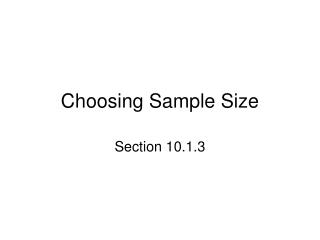DownloadDownload PresentationChoosing Sample Size

Choosing Sample Size

Download PresentationChoosing Sample Size

- - - - - - - - - - - - - - - - - - - - - - - - - - - E N D - - - - - - - - - - - - - - - - - - - - - - - - - - -
Presentation Transcript

1. Choosing Sample Size Section 10.1.3

2. Starter 10.1.3 • A coin is weighted so that it comes up heads 80% of the time. You bet \$1 that you can make it come up tails within the first 3 tosses. • Is this problem binomial, geometric, or neither? • What is the probability that you will win the bet?

3. Today’s Objective • Express confidence intervals as part of a three-phrase sentence in context • Determine how large a sample size is needed to achieve a given margin of error California Standard 17.0 Students determine confidence intervals for a simple random sample from a normal distribution of data and determine the sample size required for a desired margin of error

4. The phrasing of a C.I. • Work the problem on the slide I am about to show you. • Write a sentence that summarizes what you find in the following form: • “I am xx% confident that… • the “parameter being estimated” is… • Write this part in context • between (lower bound) and (upper bound).

5. Estimate Snickers Weights • Do snickers 1-oz “fun-size” candy bars really weigh 1 oz? • Suppose we know that the weights vary normally with σ = .005, and that the weights of 8 randomly chosen bars are .95 1.02 .98 .97 1.05 1.01 .98 1.00 • Find a 90% confidence interval for the true mean weight of fun-sized Snickers. • Write a sentence that summarizes your results using the form I just showed you.

6. Answer • The sample mean is .995 oz • Margin of error is 1.645x.005/√8=.003 oz • The C.I. is (.992, .998) • So write: “I am 90% confident that the mean weight of Snickers bars is between 0.992 oz and 0.998 oz.”

7. Sample size for margin of error • A confidence interval is formed by taking an estimate ± the margin of error • The formula for margin of error is • So if there is a certain maximum margin of error M desired, re-write as an inequality and solve for n (sample size):

8. Example • Let’s continue the example we did yesterday about the active ingredient in a painkiller. • We had three specimens and formed a 99% confidence interval in which the margin of error turned out to be 0.0101. • Recall that σ was 0.0068 • Suppose the client wants a margin of error that is no more than 0.005 g. • How many measurements do we need? • Use the formula and figure it out.

9. Answer • We still have σ = .0068 • z* = 2.576 • M has now been specified as .005 at most • So solve the inequality and state n as an integer

10. Answer • We still have σ = .0068 • z* = 2.576 • M has now been specified as .005 at most • So solve the inequality and state n as an integer

11. Answer • We still have σ = .0068 • z* = 2.576 • M has now been specified as .005 at most • So solve the inequality and state n as an integer

12. What About Proportions? • For national polling, how many people do we need to talk to in order to have a margin of error of no more than 3% in a 95% confidence interval? • Now the margin of error term becomes: m = z* √(pq/n) For purposes of this calculation, use p = 0.5 • Do the calculation now.